## What we cover

Yup tutors are experts in all K-12 math, from addition to calculus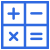### Pre-Algebra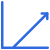### Algebra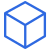### Geometry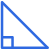### Trigonometry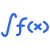### Calculus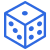### Looking for tutoring in another math subject?

We know that some schools have different naming conventions for math classes. We cover Integrated Math (Math 1, Math 2, Math 3) and all K-12 grade Common Core math. Yup can also be used for SAT and SAT II preparation. To confirm whether we cover your class or subject area, email support@yup.com.

## Pre-Algebra

Integers

Addition, subtraction, multiplication, prime factorization, exponents

Rational Numbers

Fractions, decimals, percents

Real Numbers

Absolute value, square roots, properties of addition and multiplication, scientific notation

Complex Numbers

Imaginary numbers, understanding number classifications

## Algebra

Algebra fundamentals

Properties of equality, like terms

Linear equations and inequalities

Solving linear equations and inequalities, properties of lines

Systems

Elimination, substituation, graphing, number of solutions, matrices

Functions

Properties of functions, function operations, types of functions

Polynomials

Polynomial properties, polynomial operations, Rational Root Theorem, binomial expansion

Factoring, completing the square, Quadratic Formula, parabolas

Rational functions

Simplifying rational expressions, solving rational equations, graphing rational equations

Exponents

Exponent properties

Exponentials/Logs

Graphing exponential equations, solving exponential equations, log rules, graphing logs, solving log equations

Sequences and series

Explicit and recursive formulas, geometric sequences, arithmetic sequences, sigma notation

Cartesian plane

Points, quadrants, distance and midpoint formulas

Conic sections

Properties of parabolas, ellipses, and hyperbolas

## Geometry

Coordinate Geometry

Transformations

Geometry fundamentals

Point, line, plane

Angles

Transversals, types of langles, measuring angles

Triangles

Types of triangles, triangle properties, centers of triangles, special right triangles

Similarity

Proportions

Proofs

Using postulates for proofs

2D shapes

Finding area and perimeter, regular polygons properties

3D shapes

Finding volume and surface area, properties of 3D shapes

Circles

Properties of circles, equations of circles

Constructions

Compass and straightedge constructions

## Trigonometry

Vectors

Vector operations, vector properties

Right triangle trig

SOHCAHTOA, unit circle

Trigonometric identities

Simplifying trig expressions, using identities to evaluate expressions

Graphing

Properties of trig graphs

Solving trigonometric equations

Inverse trig, solving equations on different intervals

## Calculus

Limits

Evaluating basic limits, L'Hopital's Rule

Derivatives

Derivative rules, critical points, implicit differentiation

Integrals

Finding area under a curve, u-substitution, solids of revolution

Differential Equations

Slope fields, initial value problems

## Statistics

Basic probability

Independent vs dependent, mutual exclusivity

Compound probability

Conditional probability, addition and multiplication rules, expected values

Combinatorics

Permutations, combinations

Distributions

Measures of central tendency and spread, normal distribution properties, skewness

Graphs

Scatter plots, box and whisker plots, stem and leaf plots, histograms

Correlation

Line of best fit, correlation vs causation

Inferential statistics

Testing for statistical significance, confidence intervals

Research design

Sampling, surveys, experiments, observational studies